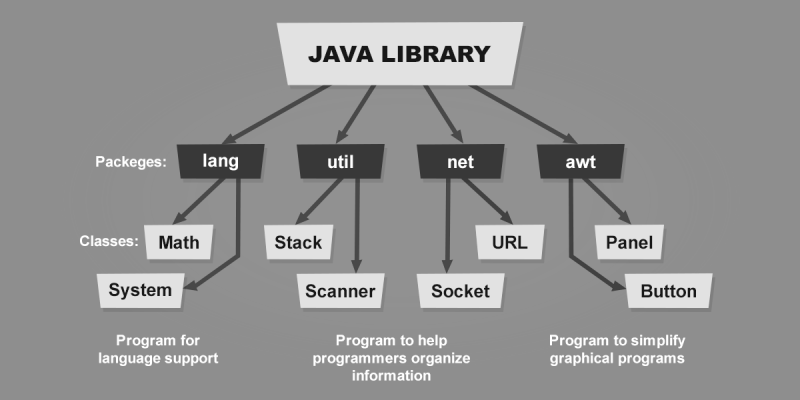# Explore hunderds of solved example topic wise.

## Java user input

### Creating a simple program

Before reading values from the user first we take a simple example and learn why user input is required. Explore the simple example to calculate the area of a circle.

To calculate the area of a circle the following steps should be followed.

2. Compute area of a circle by using the formula 3.14 * radius * radius
3. Display the result.

### Area of circle

```class ComputeArea{
public static void main(String args[]) {

//2. compute the area of a circle

//3. Display result

System.out.println("Area of circle: "+area);
}
}
```

As shown in the above example, the radius is assigned with the fixed value 20 and then the area of the circle is calculated.

The problem in the above program is that, if you run the above program for 10 times the same output will be displayed because the output is dependent on the radius that is fixed.

To make the above program interactive, we need to input the radius from the user.

Let's learn how to receive input from the user and make the above program interactive.

In Java, there are many ways to receive user input. A separate library is devoted to input and output in java. The library is called the Java IO API.

Currently, we will learn a simple way to receive user input from the user. The way to receive input from the user is the Scanner class of java.

java.util.Scanner is a class that is defined in the java library and mostly used for primitive user input in java.

Java library classes are organized in various directories and subdirectories as shown in the following figure...The library starts with a directory java then various sub-directories are there like lang, util, net, and awt as shown in the above figure. These directories and subdirectories are classed package in java.

A package is the collection of various related classes and sub-packages.

In java, a package is not a simple directory rather than a part of a class name. For example, The full name of the Scanner class in java is written as java.util.Scanner, where java is the main package, lang is the sub-package, and Scanner is the class name.

#### Creating a Scanner class object

To receive input from the user, you first need to create a java.util.Scanner class object as given below...

`Scanner input = new Scanner(System.in);`

In the above code snippet, The statement new Scanner(System.in) creates an object of Scanner type and connects it with System.in. In Java System.in refers to the standard input device that is a keyboard.

After creating a Scanner type object, it is assigned to a variable input of type scanner.

Now to read any type of primitive value from Scanner, Scanner class have method like nextInt(),nextDouble(),nextBoolean() to read integer, double and boolean type values respectively.

```double radius = input.nextDouble();
```

So the above code snippet read a double type value from the user and store it into the radius variable.

### Area of circle with user input

```import java.util.Scanner;
class ComputeArea{
public static void main(String args[]) {

//Creating a Scanner object
Scanner input = new Scanner(System.in);

//2. compute the area of a circle

//3. Display result

System.out.println("Area of circle: "+area);
}
}
```

In the above program, observe the statement import java.util.Scanner.

In java, while using any java class in your program you have to write the full name of the class. So by writing the full name of Scanner class object should be created as given below...

```java.util.Scanner input = new java.util.Scanner(System.in);
```

But if it is required to use the class name multiple times in your program, then it is difficult and cumbersome to write the full name repeatedly. So another solution is to use the import statement at the top of the program then use the class name directly as shown in the above program.